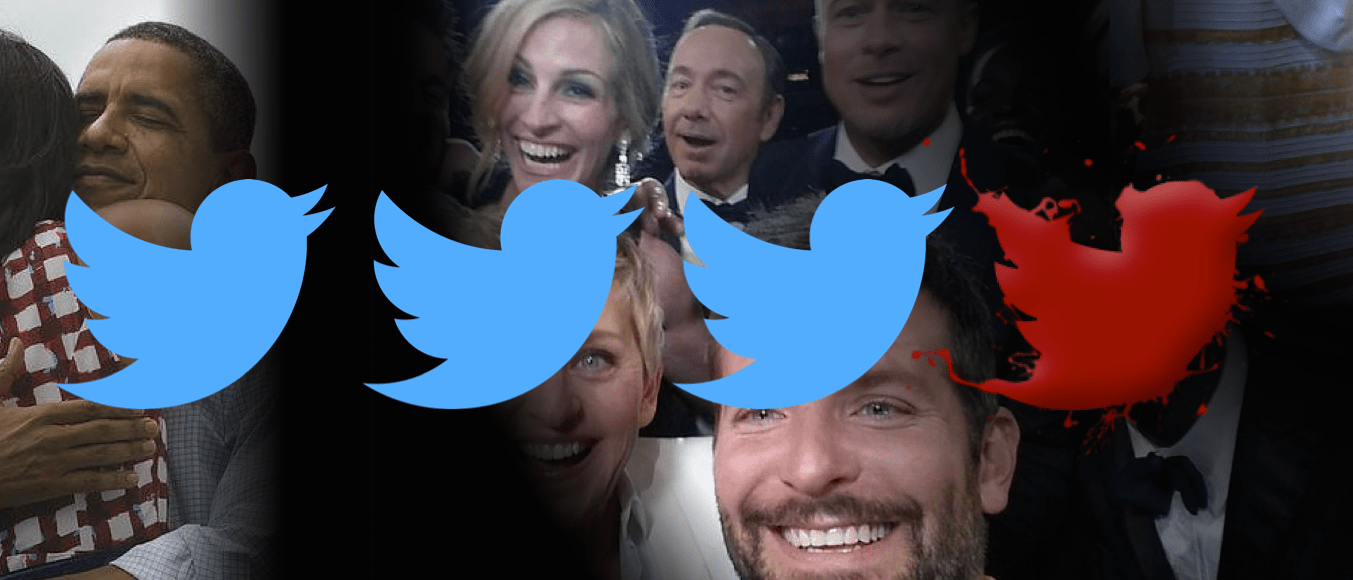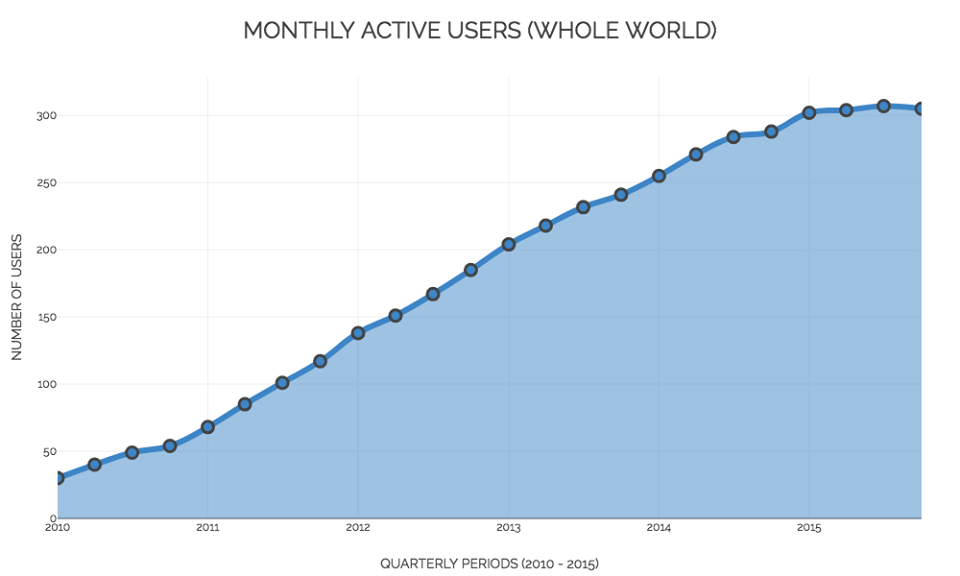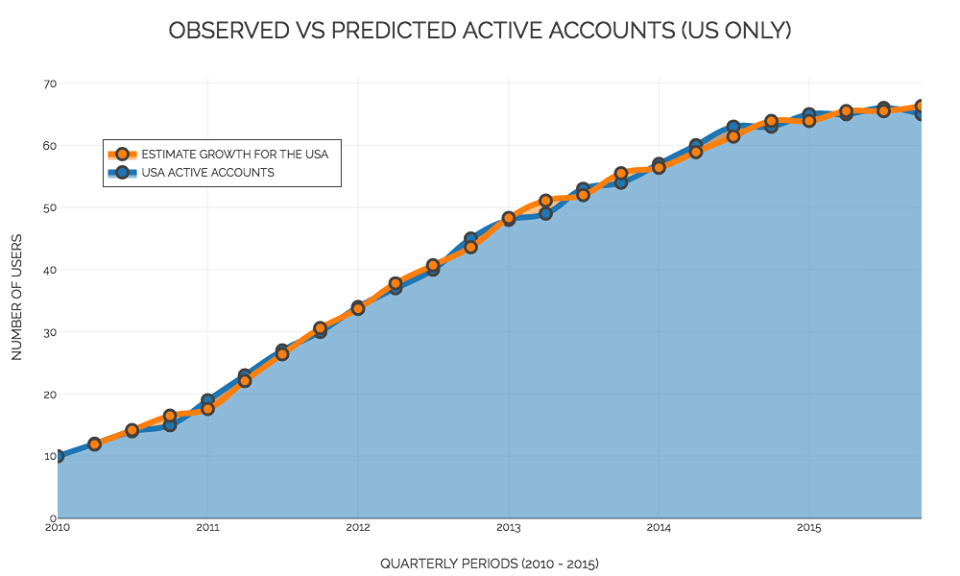# Are we getting tired of Twitter?

Here is the reason why the golden era of Twitter is goneCould one ever get tired of those 140 characters of freedom? With the ability of opening a Twitter account for free and then sharing thoughts, pictures, videos and links with the rest of the world (except for some countries), will the number of users continue to grow until everybody has an account? Recent data shows that perhaps the world is actually getting tired of Twitter: the number of monthly active users of the network, at least in the US, has practically remained constant for the last year (one could even say that it decreased slightly, taking into account the 0.7% population growth of that country). This is the end of Twitter’s golden era, in which they managed to double the amount of users year after year.

Perhaps looking at the monthly active users is not the only way to measure the performance of a social network. One could focus, instead, on the more than 500 million tweets per day, or the relevance of Twitter on social and political issues, or even the impact that a single tweet might have. However, from the business point of view, it is now a concern to its shareholders that Twitter is not attracting as many new users as it used to.Interactive version here.

Now let’s put some maths into all this. Let $U_t$ be the number of monthly active users at time $t$ and let $G_t$ be the growth of the active users in that period, so that $U_{t+1} = U_t + G_t$. Now let’s focus on $G_t$ and ask why the number of users would increase or decrease. There are two main reasons: either the active users convince their family or friends to open an account or they get bored of Twitter and stop being active users. Now, if $N$ is the number of potential Twitter users (since ok, my grandma is never going to open an account, $N$ is not really the world’s population), then $G_t$ is proportional to

$$U_t \left( \frac{N – U_t}{N}\right).$$

Hence the growth depends both on the number of active users and also on how many are not but who might eventually become active users (the expression inside the brackets). Thus we get an expression for the growth as

$$G_t = \alpha U_t \left( \frac{N – U_t}{N}\right),$$

where $\alpha$ combines the rate at which new users are convinced to join Twitter and active users get bored. We assume that $\alpha$ and $N$ are constant for a certain population group, such as the US. Using data available for the whole world, for the US only, and for outside the US, we can fit the values of $\alpha$ and $N$ for each of the three time series. The results are:

## Whole world

$N = 316.8$ million users

$\alpha = 0.2323$

## US only

$N = 67.2$ million users

$\alpha = 0.2245$

## Outside the US

$N = 250.3$ million users

$\alpha = 0.2347$.

###### NOTE: the three series were fitted separately so the estimated $N$ for the whole world is not exactly the sum of the other two series, but it’s close enough not to reject it.

It should be noted that this model does not take into account many factors, such as events that might increase the public engagement in the network (like the upcoming elections in the US), or the fact that there are some users with more than one account, or the level of internet penetration in some parts of the world and so on. However, it does provide a fairly good mathematical and statistical description of how the number of active Twitter users behave. For the US data, for example, the model provides the following prediction:Interactive version here.

This type of model (known as logistic) is frequently used in population dynamics and it has a key component: at first, the fast speed at which the population grows gives the impression of exponential growth, but then, after a certain period, the population achieves its maturity, starts growing at a much lower rate and finally stabilizes. In the long term the population will reach and then remain of size $N$. What does this mean? Bad news for the shareholders: we predict that Twitter will achieve a maximum of roughly 317 million monthly active users, from which 67 million are based in the US and 250 million in the rest of the world. Things will not get much better than that.Also, this type of model is useful in comparing the maturity of a population. If we focus on the long-term ratio (given by $U_t / N$) we can then measure the relative volume of the population, where a value closer to 1 means that the population is close to its long-term size. With the long-term ratio, we can compare two populations with different sizes, such as the number of active users from the US and the rest of the world, and the results are fascinating: the Twitter revolution started earlier in the US and by the time it reached half of its long-term size, the rest of the world was only at 40% of its maturity size. In fact, in terms of the number of active Twitter accounts, the behaviour for the rest of the world is practically the same as that of the US but with a delay of six months!Interactive version here.

The social network that sees 500 million tweets per day will continue to work as a platform to share our 140 character thoughts, reach people we couldn’t reach otherwise, help us determine whether #thedress is #blackandblue or #whiteandgold and RT the “best photo ever“. But the golden era in which Twitter doubled its number of active users every year is long gone and isn’t coming back!Rafael Prieto Curiel is doing a PhD in mathematics and crime. He is interested in mathematical modelling of any social issues, such as road accidents, migration, crime, fear and gossip.